Study Guide

# Absolute Value Functions

## Absolute Value Functions

The only absolute thing in this world is absolute value. Naturally, if absolute values are absolute, then so are absolute value functions.

Know what else is absolute? Geese. Or at least the formation that geese fly in. They conveniently always fly in a V shape. We say conveniently because absolute value graphs look like V’s too. Who knew geese knew such advanced math?

The graph y = |x| is about the simplest absolute value graph we can come across.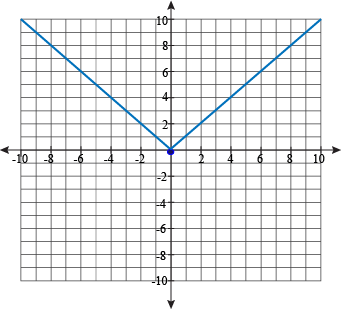Doesn't this graph look familiar? It looks exactly the same as the piecewise function that we talked about in the last unit. It should, because it's exactly the same.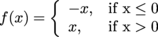In this piecewise function, f(x) is positive when x is negative, and f(x) is positive when x is positive. That f(x) just has a positive outlook on life, just like y =|x|.

For us to meet here again, it must be fate; that, or mathematics. We're leaning towards math, actually.

### Playing On Our AbsoLute

Absolute value functions can do a lot more than what we've already seen. Like play a smashing song on their lutes.

### Sample Problem

Graph y = -2|x|

A function is all well and good, but to make the graph, we need specifics. Values. Something particular to sink our teeth into and leave a mark on the page.

To that end, let's make a table of a couple values and see what pops out. Hopefully not a snake or a clown.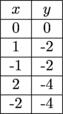Now we can make our graph.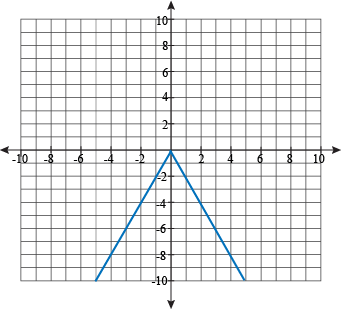The two halves of the graph come to a point at (0, 0). This is called the vertex of the absolute value function. The whole world turns on the vertex…or at least the whole function does.

We can also see that the whole graph is symmetric across the vertex, at x = 0. If we find a point on one half of the graph, we can use it to find its twin on the other half. Maybe we'll get a Lifetime movie deal out of the story of reuniting these lost siblings.

How is this graph different from y = |x|? The negative sign outside of the absolute value bars flipped the whole graph upside down. The 2 outside then made the V-shape narrower, making it easier to store in our closet. A number less than 1 would have an opposite effect, widening the graph and taking up all our closet space.

### Sample Problem

Graph y = |x + 1| + 2

From the last problem, we know that this function will be pointing up, but beyond that we're in the dark.

Ouch, did we just bump into the coffee table? Where's that light switch, mumble grumble. Wait, we think that was a table of values we bumped into.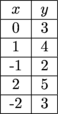Ah, much better. With the lights on, we can graph this function.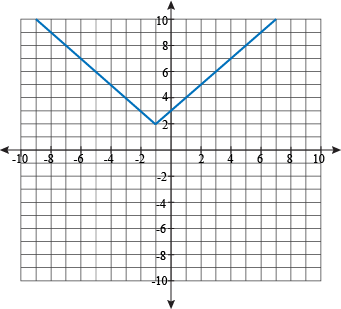Compared to plain, vanilla y = |x|, this graph has some serious flavor: it's been moved 2 up and 1 left. In general, numbers added or subtracted outside the bars move the graph up or down. Numbers added or subtracted inside the bars should order a soda if they plan on driving. They also cause the graph to move left and right, but in the opposite direction of their sign.

The symmetry of the graph has moved with the vertex; now it's symmetric about x = -1.

### V-Shaped For Victory

We can take everything we've learned and tie it together in a real math-tastic fashion.

The equation for an absolute value function has the general form:

y = a|xh| + k

It would be rather scary if we just threw this equation at you, with a bunch of letters sitting there without context. We've already worked with every piece of this equation, though, so it ain't no thang.

• The vertex of the graph is at (h, k).
• It is symmetric about the line x = h.
• The graph is V-shaped.
• It opens up if a > 0. It opens down if a < 0.
• It is wider than the graph of y = |x| if |a| < 1.
• It is narrower than the graph of y = |x| if |a| > 1.

With this information, we don't need to plot nearly as many points to make our graphs. We can find the vertex at a glance, we know which way the graph points, and we can use symmetry to find two points for the effort of finding one.

You know what that means—extra time for naps.